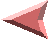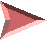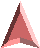# 4. Solutions for Java

There are two approaches to solving the Java hash problems. One is to ignore the hash functions supplied in the language and use something better.  This approach is taken in the appendix. Far better would be for Java to incorporate a similar solution within the language definition, thus making it a part of the language.

Individually each of the criticisms of the language or implementation can be fixed or ameliorated by the following actions:

# Criticism 1: Solution for Bad Distribution of Values

The Java implementation should use a better library hash function for all base values. Either pkphash [pearson 1990] or buzhash [uzgalis 1990] suitably modified to produce a 64-bit hash would be the best.

# Criticism 2: Solution for Too Small a Hash Range.

The Java `hash.Code` method currently returns an `int` (defined as 32-bits). This should at least be changed to a positive or unsigned long. Better yet it should return an unsigned long of type `Hash`.

Note the % operator is only partially defined in many programming languages.  Usually the definition excludes the meaning of the operator for negative values of its arguments. The meaning of % can, of course, be explicitly defined, in the Java specification it should be.  But it is common practice in other programming languages to leave the meaning of % only partially defined so that implementations can use efficient ways to code it depending on how the machine arithmetic works. Java's choice of defining hash values as signed numbers forces the programmer into understanding an obscure area of machine arithmetic that is best left untouched. By defining % on a special Hash type, one could restrict the denominator to be positive, and by keeping the Hash value unsigned, all these problems go away.

# Criticism 3: Solution for No Hash Value Operations.

Java should define a new abstract type called Hash with operations for constructing, operating on, and casting Hash values into the integer types.

One may ask why create a new type just for hashing?  Surely algorithms manipulating hash values are not common enough to make this a necessity? First, a hash algebra structures and delimits the ideas of hash value manipulation, guiding a programmer in the right direction.  Second, it reduces the chance of making mistakes.  Third, while the algebra is simple it may not be obvious, so encoding it in a formal abstract syntactic structure presents the ideas clearly.  Fourth, and maybe the most important reason is that there is no integer operation that combines two Hash values sufficiently well.  By defining a hash type one can add the necessary operation.

Eight methods or operators are useful for Hash values. One method takes keys (of arbitrary type) and produces a Hash. Four methods take Hash values as inputs and yield Boolean values. Two methods take Hash values as inputs and yield Hash values as outputs, and one method takes a Hash value and an integral value and produces an integer bin number. Each of these methods and operations are described in more detail below both in the chart and in written form after the chart.  A possible scheme for implementation is given after the phrase `code:' in the table. The appendix contains a Hash Java class implementation of this idea; it would be far more general and could be more efficient if the Hash algebra was built into the Java language as primitives.

In the following table identifiers are named by their type so: hash1, hash2, hashr name values of the type Hash (which is implemented as a 64 bit unsigned integer). In a similar way I have encoded type information in other identifier names in the descriptions below. The identifier key can be of any type, one only needs to know its length in bits or bytes.  In the table, the arrow symbol () represents `yields'.

 HASH A KEY: key.hashCode() hashr code: hashr = hash(key) alternatively: where hash is a library hash function that #key hashr returns a 64-bit unsigned integer hash value. NEXT HASH: hashkey.hashCode() hashr code: hashr = hash(hashkey) #hashkey hashr This is really the same operation as Hash a Key. HASH COMPARISONS: hash1 = hash2 boolr code: boolr = hash1 == hash2; hash1 != hash2 boolr code: boolr = hash1 != hash2; hash1 < hash2 boolr code: boolr = hash1 < hash2; hash1 > hash2 boolr code: boolr = hash1 > hash2; WEAK AGGLOMERATION: hash1 + hash2 hashr code: hashr = hash1 XOR hash2; STRONG AGGLOMERATION: hash1 * hash2 hashr code: hashr = hash( cat(hash1,hash2) ); The cat creates a 128-bit value from the concatenation of the two 64-bit arguments. REDUCE HASH TO INTEGER: hash1 % long2 longr code: if ( long2 <= 0 ) then error; longr =  hash1 % long2; This 64-bit integer can be cast to any other integer type.

 Abstract Algebra of Hash Values

The formal properties of a similar algebra are described in [tong97]. An exploration of ideas that might provide a theoretical foundation for hashing will be given in [tong96].

An implementation of the abstract algebra in Java is given in the appendix. It can't use a compact operator syntax because it is a Java user defined class which is not allowed to define operators. However it does define methods in a Hash class based on buzhash that could be used as an alternative to the Java-provided hash.

The HASH A KEY operation should cause the key to be hashed and a characteristic hash value to be created. The key can be of arbitrary type, but the length of the key must be known.

Since Java does not allow operator definitions, this method is called using a Java `Hash` constructor as shown in the code in the appendix. Constructors can't be invoked as `string1.hashCode()` either, since the Hash method won't be found in the Java String class, that will produce an invocation of the Java language defined hash. So to get a Hash value from a String one must use ```new Hash(string1)``` causing a Hash value to be created which is the hash of `string1`.

A special case of this Hash constructor operation creates a Hash value from another Hash value.  That is the hash of a hash. Successive uses of hashing a hash will produce a sequence of hash values all related to the original key value. This is written in Java as: `new Hash(hx)`. This is useful for multiple hashing in a variety of algorithms. For example it could be used to produce the ten hashes based on the word that the Bell Labs Unix spell program [bently86, mcilroy82] does to check spelling.  This works because hash clashes in a 64-bit domain are so rare as to be non-existent, clashes occur more frequently after the range is reduced to some implementation table size, but this still leaves the hashes and its nine rehashings at random places in the output range.

HASH COMPARISON methods allow using a 64-bit hash as an alias for its key. This allows one to create, for example, a balanced binary search tree without balancing the tree [uzgalis94].  That is, for any algorithm that requires random input values, it allows the cheap creation a random alias hash that will satisfy the assumption.  Many algorithms require comparing keys thus comparison operations are provided as a part of the Hash class.

One can, of course, implement all six standard comparisons, only four are shown, the others are trivial extensions.

WEAK AGGLOMERATION allows constructing progressive hash values which can have component hashes deleted from them. Part of the algebra of weak agglomeration states:

 #x + #x = 0 #x + #y = #y + #x #x + #y + #x = #y

(where the unary operator # is the hash operator, and the
x and y are arbitrarily typed keys.) Weak agglomeration is useful in searching for phrases, where to create the hash value of the current phrase one adds the hash of the next word and removes the hash of the oldest word.

Weak agglomeration operates only on corresponding bits of the hash arguments, and it affects the same bit of the resulting hash: a 1-bit parallel agglomeration. This operation may not mask correlation between the two argument hash values and thus on the average it may bias the distribution of the result. If you assume the hash function did a clean job of hashing the original keys and the argument keys are independent of each other, then weak agglomeration will maintain the uniform random distribution of these combined values. If the argument keys were highly correlated then using weak agglomeration is not recommended.

This method is called `wag` in the code in the appendix. This is invoked as `hx.wag(hb)` causing a Hash value which is the weak agglomeration of hashes `hx` and `hb` to be returned.

STRONG AGGLOMERATION guarantees a better hash value distribution, it will be insensitive to key correlations, and provide a uniform random distribution of keys, but the resulting hash is inseparable and non-commutative. It is a n-by-n-bit agglomeration, where each bit of the two inputs affects each bit of the output hash value. This is useful for constructing a new hash value for a class value.

This method is called `sag` in the code in the appendix. This is invoked as `hx.sag(hb)` creating a Hash value which is the strong agglomeration of the hashes `hx` and `hb` to be returned.

As an example, assume a new class defining employee information and it constructs the employee identification from three elements: an integer element giving the department called dept, another integer element giving the year of birth of the employee, birth_yr, and the last an integer sequence number which goes up by one for each department, seq. Then one can get a combined hash value for the whole key with the following expression:

 hash_code = #dept * #birth_yr * #seq; or its somewhat more verbose Java equivalent using the Hash class hash_code = ((new Hash(dept)).sag.(new Hash(birth_yr))) .sag(new Hash(seq));

REDUCE TO LONG produces long integers to use as an index from a hash value. This is best represented as the % (mod) operator.  A guard against negative numbers should be in place for the operation.  That is it should throw a new division-by-negative-number exception.  The value produces varies from 0 to n-1, where n is the denominator of the %.

This method is called `bag` in the code in the appendix. The Java class code in the appendix avoids the negative number problems by forcing both the numerator and denominator to be positive.

As an example it is invoked as `hx.bag(100)` creating a integer value in the range 0 to 99 from hash `hx`.

# Criticism 4 Solution for No Defined Hash Algorithm in the Specifications

The hash function currently supplied with the Java implementation is next to useless and the Java specification fails to provide any invariants one might use to program it.

The Java language specification should define a general library hash function so that hashing works uniformly across the network and all implementations. This means defining the function precisely in the specification. Currently there are only a few known algorithms good enough to be a library function. It should define the hash function as one of the good library functions given in this paper, either pkphash or buzhash.

Java should by default hash any class (base or user defined) using this same function.  The hash of a base class is the hash algorithm applied to all the bits of the class.  The hash of a user defined class should be applied across all non-reference bits in the class.  The user can override the system supplied hash function in a new class by defining his own if hashing for this class should be done on a different subset of the class values, or because it needs some other characteristic.Copyright © 1996 Robert Uzgalis, All Rights Reserved.
Contact: Robert Uzgalis <buz@zis.com>
home

This paper was automatically translated from troff into html by t2h.